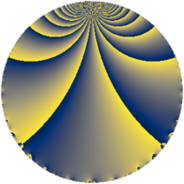# Properties

 Label 48.2.jLevel $48$ Weight $2$ Character orbit 48.j Rep. character $\chi_{48}(13,\cdot)$ Character field $\Q(\zeta_{4})$ Dimension $8$ Newform subspaces $1$ Sturm bound $16$ Trace bound $0$

# Related objects

## Defining parameters

 Level: $$N$$ $$=$$ $$48 = 2^{4} \cdot 3$$ Weight: $$k$$ $$=$$ $$2$$ Character orbit: $$[\chi]$$ $$=$$ 48.j (of order $$4$$ and degree $$2$$) Character conductor: $$\operatorname{cond}(\chi)$$ $$=$$ $$16$$ Character field: $$\Q(i)$$ Newform subspaces: $$1$$ Sturm bound: $$16$$ Trace bound: $$0$$

## Dimensions

The following table gives the dimensions of various subspaces of $$M_{2}(48, [\chi])$$.

Total New Old
Modular forms 20 8 12
Cusp forms 12 8 4
Eisenstein series 8 0 8

## Trace form

 $$8 q - 4 q^{4} - 12 q^{8} + O(q^{10})$$ $$8 q - 4 q^{4} - 12 q^{8} - 8 q^{10} - 8 q^{11} + 8 q^{12} + 12 q^{14} - 8 q^{15} + 4 q^{18} - 8 q^{19} + 16 q^{20} + 4 q^{24} + 20 q^{26} + 8 q^{28} - 16 q^{29} - 8 q^{30} + 24 q^{31} + 24 q^{35} - 4 q^{36} - 16 q^{37} - 8 q^{38} + 16 q^{40} - 20 q^{42} - 8 q^{43} - 40 q^{44} - 8 q^{46} - 16 q^{48} - 8 q^{49} - 36 q^{50} + 8 q^{51} - 16 q^{52} + 16 q^{53} + 4 q^{54} - 16 q^{58} + 32 q^{59} + 24 q^{60} + 16 q^{61} - 12 q^{62} + 8 q^{63} + 8 q^{64} - 16 q^{65} + 24 q^{66} - 16 q^{67} + 32 q^{68} + 16 q^{69} + 32 q^{70} - 4 q^{72} + 52 q^{74} + 16 q^{75} + 8 q^{76} + 16 q^{77} - 12 q^{78} - 24 q^{79} + 8 q^{80} - 8 q^{81} + 40 q^{82} - 40 q^{83} - 24 q^{84} - 16 q^{85} - 16 q^{86} + 32 q^{88} - 8 q^{90} - 8 q^{91} - 16 q^{92} + 8 q^{94} - 48 q^{95} - 40 q^{98} - 8 q^{99} + O(q^{100})$$

## Decomposition of $$S_{2}^{\mathrm{new}}(48, [\chi])$$ into newform subspaces

Label Dim $A$ Field CM Traces $q$-expansion
$a_{2}$ $a_{3}$ $a_{5}$ $a_{7}$
48.2.j.a $8$ $0.383$ 8.0.18939904.2 None $$0$$ $$0$$ $$0$$ $$0$$ $$q+(-\beta _{4}+\beta _{5})q^{2}-\beta _{5}q^{3}+(-1+\beta _{2}+\cdots)q^{4}+\cdots$$

## Decomposition of $$S_{2}^{\mathrm{old}}(48, [\chi])$$ into lower level spaces

$$S_{2}^{\mathrm{old}}(48, [\chi]) \cong$$ $$S_{2}^{\mathrm{new}}(16, [\chi])$$$$^{\oplus 2}$$